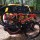## Convert an integer to a sequence of digits in Xquery

I am new to the functional programming paradigm and Xquery. As part of a larger exercise, I wanted to sum the digits of an integer.  The first part of that problem is to solve the smaller problem: convert an integer to a sequence of integers that I can then pass to fn:sum as a parameter.

```(: I've declared the function in the nwnu namespace for testing purposes :)
declare function nwnu:number-to-seq(\$num as xs:integer)
as xs:integer* {
if(\$num gt 9) then
(
nwnu:number-to-seq(xs:integer(math:floor(\$num div 10))),
xs:integer(math:floor(\$num mod 10))
)
else
\$num
};```

We’ll employ a recursive strategy to build our sequence.  First, we’ll check whether or not the number is greater than 9.  If the number is already a single digit, then we’ll just return it into the sequence since that is ultimately what we are adding to the sequence anyway.

Otherwise, we’ll add the the remainder of dividing the number by 10 with the modulus operator; this will isolate the right-most digit in the integer.  We’ll then recursively call our function with the rounded down result of dividing the input with 10.  So, for the initial input 1250, we will recursively call the function on:

`1250 -> 125 -> 12 -> 1`

### Testing the function

I am using Xquery with the MarkLogic engine.  If you have a properly configured AppServer, you can test the function with the following script.

```xquery version "1.0-ml";
import module namespace nwnu = "https://nelsonwells.net/xquery/number-utils"
at "/lib/number-utils.xqy";
let \$seq := (13857, 1847471, 11183, 44819)
let \$out := for \$i in \$seq
return
<item>
<input>{\$i}</input>
<output>{nwnu:number-to-seq(\$i)}</output>
</item>
return <items>{\$out}</items>```
1.Carlos Roque